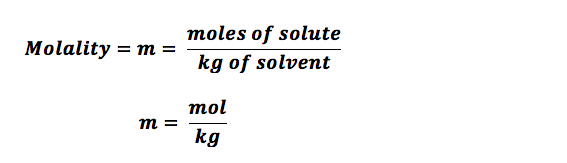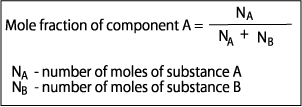Resources:

Have you found videos, websites, or explanations that helped you understand this chapter? Let us know and we'll add them to "Resources" part of this page for other students to use.

Question 1

Common commercial acids and bases are aqueous solutions with the following properties: HCl, density 1.19 g/cm3 and 34% solute by mass. Calculate the molarity. (1mL = 1 cm3)Explanation1.19 g/cm3  ---> 1190 g / L

( 1190 g / L ) x ( 34g /100g ) = 404 g/ L

(404 g/ L) / (36.46 g HCL/ mol) =  11.09 mol/ L

Question 2

Common commercial acids and bases are aqueous solutions with the following properties: nitric acid (HNO3), density 1.42 g/cm3 and 65% solute by mass. Calculate the molarity.Explanation1.42 g/cm3  ---> 1420 g / L

( 1420 g / L ) x ( 64g /100g ) = 908.8 g/ L

(908.8 g/ L)  / (63 g HNO3/ mol) =  14.7 mol/ L

Question 3

Common commercial acids and bases are aqueous solutions with the following properties: HCl, density 1.19 g/cm3 and 31% solute by mass. Calculate the molality.Explanationmoles HCl = 31 g / (36.461 g/mol)  = 0.850 mol
mass water = 100 - 31=" 69" g = 0.069 Kg
m = .850 / 0.069 =12.32 mol / kg

Question 4

Common commercial acids and bases are aqueous solutions with the following properties: nitric acid (HNO3), density 1.42 g/cm3 and 67% solute by mass. Calculate the molality.Explanationmoles HNO3= 67 g / ( 63 g/mol)  =1.06 mol
mass water = 100 - 67=" 33" g = 0.033 Kg
m = 1.06 / 0.033 = 32.21 mol / kg

Question 5

Common commercial acids and bases are aqueous solutions with the following properties: HCl, density 1.19 g/cm3 and 31% solute by mass. Calculate the mole fraction.Explanationmoles HCl = 31g / (36.461 g/mol) = 0.850 mol
mass water = 100 - 31 = 69 g
moles water = 69 g/ 18.02 g/mol= 3.82 mol
mole fraction HCl = .850 / (0.850 + 3.82) = 0.18

Question 6

Common commercial acids and bases are aqueous solutions with the following properties: nitric acid (HNO3), density 1.42 g/cm3 and 61% solute by mass. Calculate the mole fraction.Explanationmoles HCl = 61 g / (63 g/mol) = 0.968 mol
mass water = 100 - 61 = 39 g
moles water = 39 g/ 18.02 g/mol= 3.385
mole fraction HCl = .968 / (0.850 + 3.82) = 0.31

Question 7

On the basis of the nature of the intermolecular attractions, arrange water, ethanol (C2H5OH), and chloroform (CHCl3) in order of decreasing solubility in carbon tetrachloride (CCl4).

Select one:Explanation

• Nonpolar with nonpolar (like dissolves likes)
• CHCl = nonpolar
• C2H5OH = polar  (only one polar bond O-H)
• H2O = polar (two polar bonds O-H )

Question 8

Predict the relative solubility of each compound in the two solvents on the basis of intermolecular attractions. Is NaCl more soluble in water or in carbon tetrachloride?

Select one:Explanation

• NaCl is ionic compound (polar) that completely dissolves in water

Question 9

Is iodine (I2) more soluble in water or in benzene?

Select one:Explanation

• Iodine (I2) is nonpolar so it dissolves in a nonpolar solution C6H6. (like dissolves like)

Question 10

Is ethanol (C2H5OH) more soluble in water or in hexane?

Select one:Explanation

• Ethanol (C2H5OH)  is polar so it dissolves in polar solution (

Question 11

Is ethylene glycoL (HOCH2CH2OH) more soluble in ethanol or in benzene?

Select one:Explanation

• Ethylene glycoL (HOCH2CH2OH) is a polar so it dissolves in a polar solution ( ). The O-H bond makes any molecule polar.

Question 12

Check the correct statements regarding solubilities in liquids. (The following figure for question (c) shows the solubility of some ionic compounds as a function of temperature.)Select one or more:Explanation

Rule of Thumb: As temperatures increase gases decrease in solubility

The steeper slope the greater solubility with increase in temperature.

Question 13

At 25 oC and 2.0 atm, the enthalpy of solution of neon in water is -2.46 kJ/mol, and its solubility is 9.07 x 10-4 m. Check the conditions for which the solubility of neon is greater than 9.07 x 10-4m.

Select one or more:Explanation

Low temp and high pressure increases the solubility of Ne.

Question 14

The enthalpy of solution of nitrous oxide (N2O) in water is -12 kJ/mol and its solubility at 20 oC and 1.00 atm is 0.121 g per 100. g of water. Calculate the molal solubility of nitrous oxide in water at 0.800 atm and 20 oC. Hint, first find Henry's law constant at 20 oC and 1.00 atm.

Hint given in feedbackExplanation

Solubility = 0.121 g per 100 g of water

Assuming density of water ≈ 1 g/mL, volume of solution = 100 mL

Concentration, c (molarity) = mass * 1000 / (molar mass * volume of solution)

= 0.121 g * 1000 / (44.01 g/mol * 100 mL)

= 0.0275 M

Pressure, P = 1.00 atm

Henry's law constant, k = c/ P

k = 0.0275 M/ 1.00 atm

k = 0.0275 M/atm

Solubility, c = k * P

= 0.0275 M/atm * 0.800 atm

= 0.022 M

Question 15

A 0.511 g sample of a nonvolatile, yellow crystalline solid dissolves in 13.0 g of benzene, producing a solution that freezes at 5.39 oC. Find the molar mass of the yellow solid. The following may be useful: The freezing point of benzene is 5.51 oC and the freezing point depression constant, kf, is 4.90 oC/m.

Hint given in feedbackExplanation

Procedure:
(1) Solve ΔTf = mkf for m and find m.
(2) Recall m = mol solute/ kg solvent.
(3) Multiply m by kg solvent to get moles solute (yellow solid).
(4) Recall the definition of molar mass is g/mol. Simply divide the mass of the solute by the number of moles this mass corresponds to.

Δt = i Kf m

Pure benzene freezes at 5.51 °C

Δt = 5.51 -5.39 = 0.12

0.12 °C = (1) (4.9°C kg mol-1) (x / 0.013 kg)

x = 3.183 x 10-4 Mol

Molar mass of the compound = 0.511 g/ 3.183 x 10-4 mol = 1605 gm/mol

Question 16

A 4.60% solution by mass of an enzyme in water has an osmotic pressure of 17.9 torr at 286 K. Calculate the molar mass of this enzyme. (Assume that the density of the solution is 1.00 g/mL.)

Hint given in feedbackExplanation

Procedure:
(1) Solve π = MRT for M and find M.
(2) Recall M = mol solute/L solution.
(3) Multiply M by L solution to get moles solute.
(4) Recall the definition of molar mass is g/mol. Simply divide the mass of the solute by the number of moles this mass corresponds to.

M = π / RT

R = 62.36367 L torr/K mol

M = 17.9 / ( 62.36*286) = 0.0010036 mol/L

.0460g x 1g/ml x 1000ml/L = 46 g/L

( 46 g/L) / ( 0.0010036 mol/L  ) = 45832.86 g/mol = 46000 g/mol

Question 17

A saline solution contains 6.1 g of NaCl in 1.00 kg of water. Assuming an ideal value for the Van't Hoff factor, i, calculate the freezing point of this solution in oC.Explanation6.1 g of NaCl

6.1g/ (58 g/mol NaCl) = 0.10436 mol NaCl

m =  0.10436 mol / 1kg =  0.10436

K = 1.86 (water)

i = 2 (NaCl dislocates into two ions)

T f = (0.10436) (1.86) (2) = 0.388

0 -  0.388 = -0.388

Question 18

A saline solution contains 7.1 g of NaCl per 0.80 liter of solution. Assuming an ideal value for the van't Hoff factor, i, calculate the osmotic pressure (in atm) of this solution at 291 K.Explanationi = 2 (NaCl dislocates into two ions)

R = .08206
T = 291 K
M = molality (mol / L)

7.1 g NaCl / (58.45 g/mol NaCl) = 0.1215 mol NaCl

0.1215 mol NaCl /  0.80L = 0.15187

π = 2 x 0.15187 x  .08206 x 291 K = 7.25 torr

Question 19

A reverse osmosis unit is used to obtain drinkable water from a source that contains 0.600 g NaCl per liter. What is the minimum pressure (in torr) that must be applied across the semipermeable membrane to obtain water? Assume room temperature or about 298 K. (Don′t forget the van′t Hoff factor!)Explanationi = 2 (NaCl dislocates into two ions)

R = 62.364 L Torr mol-1K-1,

T = 298 K

M = (.6 g/L) x (1 mol/ 58.45g NaCl) = .010265 mol/L

π = 2 x .010265 mol/L x 62.364 x 298 K = 381 torr

Question 20

When 0.030 mol of HCl dissolves in 100.0 g of benzene, the solution freezes at 4.04 oC. When 0.030 mol of HCl dissolves in 100.0 g of water, the solution freezes at -1.07 oC. Use data in the following table to calculate the expected molality of HCl in each solvent, ignoring the van't Hoff factor. Check the 3 statements which are correct.Select one or more:Explanationdelta T in benzene is 5.50 - 4.0 = 1.5 C

molality is (0.03 mol / 0.1 kg) = 0.3 m

1.5 = (5.12)(0.3)(1)

The van't Hoff factor is equal to 1 which means that HCl does NOT dissociate in benzene.

delta T in water is 1.07 C
molality is still 0.3 m

1.07 = (1.86)(0.3)(2)

The van't Hoff factor is equal to 2 which means that HCl DOES dissociate in water.

Question 21

A mixture contains 25 g of cyclohexane (C6H12) and 44 g of 2-methylpentane (C6H14). The mixture of liquids is at 35 oC . At this temperature, the vapor pressure of pure cyclohexane is 150 torr, and that of pure 2-methylpentane is 313 torr. Assume this is an ideal solution. What is the mole fraction of cyclohexane in the liquid phase?ExplanationC6H12 = 25g / (84 g/mol) = 0.2976 mol

(C6H14) =  44g / (86 g/mol) =  0.5116 mol

0.2976 / (0.2976 + 0.5116) = 0.3677

Question 22

What is the vapor pressure (in torr) of cyclohexane above the solution?Explanation

P cyc vapor= x P cyc liquid

P cyc  vapor0.3677 (150)

P cyc vapor= 55.5

Question 23

What is the mole fraction of cyclohexane in the vapor phase?Explanation

Calculate Pi for C6H14 first

P(C6H12) = (313 torr) (1 - 0.3677) = 197.9 torr.

55.5 / (55.5 + 197.9) = 0.22

Question 24

At a pressure of 760 torr, formic acid (HCOOH; boiling point = 100.7 oC) and water (H2O; boiling point = 100.0 oC) form an azeotropic mixture, boiling at 107.1 oC, that is 77.5% by mass formic acid. At the boiling point of the azeotrope (107.1 oC), the vapor pressure of pure formic acid is 917 torr, and that of pure water 974 torr. If the solution obeyed Raoult's law for both components, what would be the vapor pressure (in torr) of formic acid at 107.1 oC?Explanation

77.5 g /  (45g/mol HCOOH) = 1.68

22.5 g/ (18.02g/ mol H2O) = 1.25

mole fraction of acid:  1.68 / (1.25+1.68) = 0.573

P acid vapor  = x P acid liquid

P acid vapor  = (0.573) x (917 torr)

P acid vapor  = 525.79 torr

Question 25

If the solution obeyed Raoult's law for both components, what would be the vapor pressure of the water (in torr) at 107.1 oC?Explanation

mole fraction of water: 1- 0.573 = 0.427

P acid vapor  = x P acid liquid

P acid vapor  = (0.427) x (974torr)

P acid vapor  = 415.53 torr

Question 26

If the solution obeyed Raoult's law for both components, what would be the total vapor pressure (in torr) at 107.1 oC?Explanation

P total = P acid + P water

P total 525.79 torr415.53 torr

P total = 941.32 (theoretical)

Question 27

Check those statements which are true regarding the above azeotropic mixture.

Select one or more:ExplanationThe calculated pressure is the theoretical value. The actual value is less.

A negative deviation means actual value is less than the theoretical. This means the solutes and solvents have a strong bond.